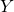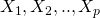## Assumptions for linear regression

Linear regression is one of the most commonly used statistical methods; it allows us to model how an outcome variabledepends on one or more predictor (sometimes called independent variables). In particular, we model how the mean, or expectation, of the outcomevaries as a function of the predictors: